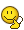# Find integral of fraction

songoku
Homework Statement:
Find the integration of .... (for complete question see "attempt at solution")
Relevant Equations:
Integration
Find integration of:
$\frac {1}{(x+1)(x^2 + x -1)^\frac{1}{2}}$

What I did:
1. Use completing square method for the term inside the square root

2. Use trigonometry substitution (I use secan)

3. After simplifying, use another trigonometry substitution (I use weierstrass substitution)

4. Use another trigonometry substitution (I use tan)

My working was so long and taking too much time. Is there any other way to solve this integration?

Thanks

Mentor
2022 Award
Can you bring it into the form ## \dfrac{f'(x)}{1+f(x)^2}## where ##f(x)## is the quotient of a polynomial in ##x## and the root ##\sqrt{x^2+x-1}## in the denominator? Find ##f(x)## and then you get ##\tan^{-1} f(x)## as antiderivative (up to a sign).

•songoku and Delta2
Homework Helper
Gold Member
I am afraid this integral has no easy route to calculate it...

Unless of course someone whispers you "Psst, this integral is of the form ##\tan^{-1}f(x)## " in which case you could try to find f(x) as suggested in post #4.

•songoku
songoku
I am afraid this integral has no easy route to calculate it...

Unless of course someone whispers you "Psst, this integral is of the form ##\tan^{-1}f(x)## " in which case you could try to find f(x) as suggested in post #4.

Unfortunately even though I heard the whisper I still can not do anythingCan you bring it into the form ## \dfrac{f'(x)}{1+f(x)^2}## where ##f(x)## is the quotient of a polynomial in ##x## and the root ##\sqrt{x^2+x-1}## in the denominator? Find ##f(x)## and then you get ##\tan^{-1} f(x)## as antiderivative (up to a sign).

Sorry I can not. You mean I have to find f(x), which is the quotient of a certain polynomial (let say g(x)) divided by x2 + x - 1?

g(x) : (x2 + x - 1) and the quotient of this long division is f(x)? Before finding f(x), I need to find g(x) first?

Thanks

Mentor
2022 Award
Well, WolframAlpha has the solution: something like ##-\arctan \dfrac{x+a}{b\sqrt{x^2+x-1}}##, so the reverse inspection, i.e. the derivative of ##\arctan## points the way.

I thought about the Weierstraß substitution ##t=\tan \frac{x}{2}## which is normally used to turn trigonometric integrands into polynomials, and apply it the other way around, but it didn't look promising. So I have no idea what the best purely forward path is.

•songoku
songoku
ok. thank you very much for the help fresh_42 and delta2

•Delta2
Fred Wright
Considering the substitutions your text as armed you with so far, I suspect that there may have been a misprint in your problem assignment and the integration is actually$$\int \frac {dx} {(2x + 1) \sqrt {x^2 + x -1}}$$